Home > INT2 > Chapter 4 > Lesson 4.1.2 > Problem4-18

4-18.

Use the process you developed in problem 4-13 to factor the following quadratic expressions. Homework Help ✎

1. $x^2-4x-12$

Fill in the rest of the diagram. Remember that the product of the diagonals must be equal.

$(x−6)(x+2)$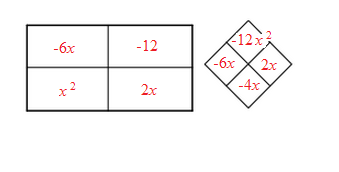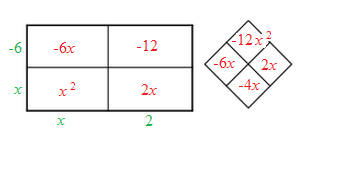2. $4x^2+4x+1$

$(2x+1)^2$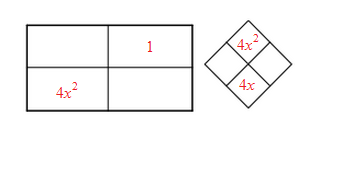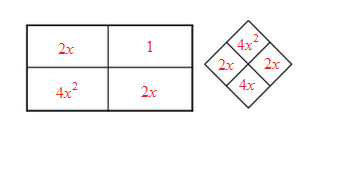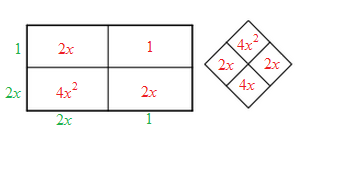3. $2x^2-9x-5$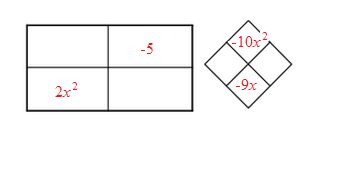4. $3x^2+10x-8$